# 第一章 绪论

## 1.1 基本术语：

eg.总共100个西瓜

eg.西瓜的特征：色泽、根蒂、敲声等

eg.西瓜的色泽有青绿、乌黑等。
eg.属性空间是属性值的全体集合，比如西瓜的三个属性（色泽、根蒂、敲声），张成3维空间，每个西瓜都在该空间中找到自己的坐标，每个点也对应一个"坐标向量",也称"特征向量"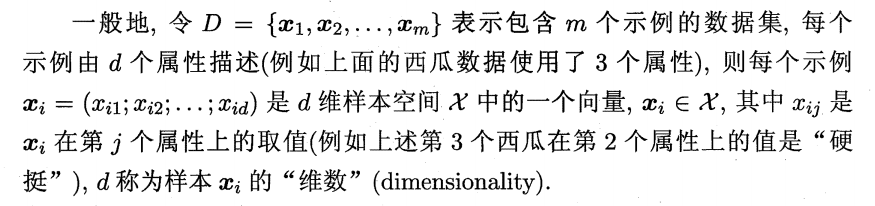比如：分类+回归

比如：聚类

狭义：从训练数据中得到概念
广义：从样本中学习

引导算法确立偏好的一般性原则：

学习算法的期望性能相同：

# 第二章 模型评估与选择

## 2.1 经验误差与过拟合

$$1-a/m$$

## 2.2 评估方法

### 1.留出法(hold-out)

"留出法"直接将数据集D划分为两个互斥的集合,其中一个集合作为训练集S,另一个作为测试集T. $$D=S∪T,S∩T=∅$$.在S上训练出模型后，用T来评估其测试误差，作为对泛化误差的估计.
分层采样：保留类别比例的采样方式。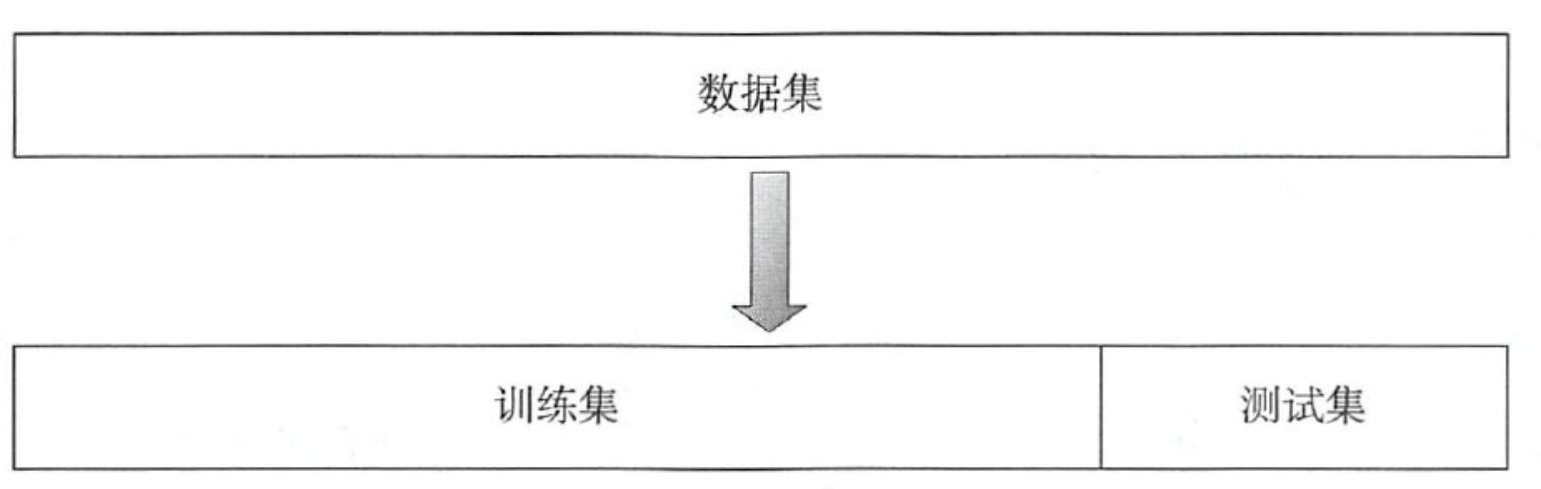### 2.交叉验证法(cross validation)/k折交叉验证(k-fold cross validation)

"交叉验证法" (cross alidation) 将数据D分为k个大小相似的互斥子集,$$D= D1∪D2∪...Dk, Di∩Dj = ø(i≠j)$$,每个子集Di尽可能保持数据分布的一致性，即从D通过分层采样得到。然后，每次用k-1 子集的并集作为训练集，余下的那个子集作测试集;这样就可获得k组训练/测试集，从而可进行k次训练和测试,最终返回的是k个测试结果的均值。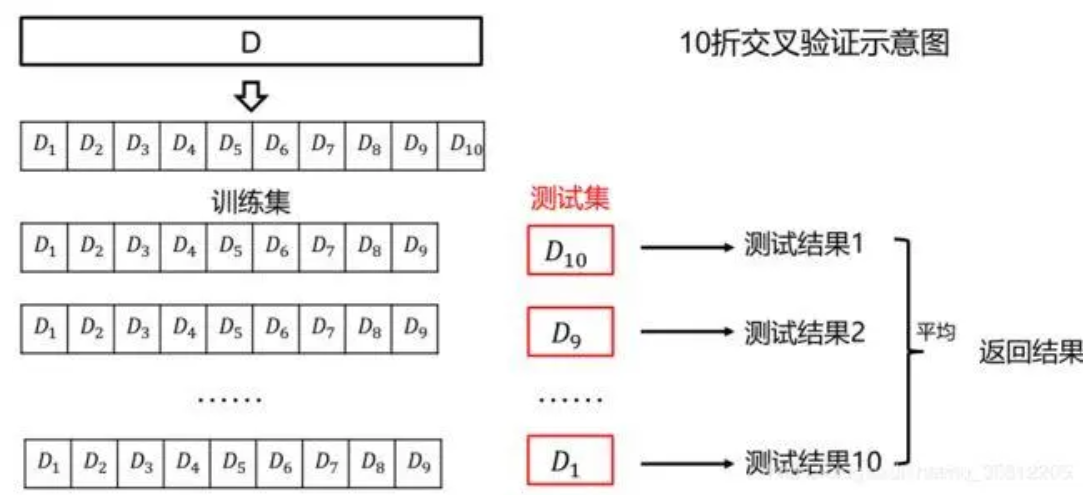假定数据集D中包含 m样本，令 k=m 则得到了交叉验证法的一个特例:留一法(Leave-One-Out，简称LOO).显然，留一法不受随机样本划分方式的影响，因为m个样本只有唯一的方式划分为m个子集一一每个子集包含一个样本;留一法使用的训练集与初始数据集相比只少了一个样本，这就使得在绝大多数情况下，留一法中被实际评估的模型与期望评估的用D训练出的模型很相似.因此，留一法的评估结果往往被认为比较准确.

### 3.自助法(bootstrapping)

“自助法”以自助采样法(bootstrap sampling)为基础.给定包含m个样本的数据集D,我们对它进行采样产生数据集D': 每次随机从D中挑选一个样本，将其拷贝放入D',然后再将该样本放回初始数据集D中，使得该样本在下次采样时仍有可能被采到；这个过程重复执行m次后，我们就得到了包含m个样本的数据集D',这就是自助采样的结果。

自助法在数据集较小、难以有效划分训练/测试集时很有用;此外，自助法能从初始数据集中产生多个不同的训练集，这对集成学习等方法有很大的好处.然而，自助法产生的数据集改变了初始数据集的分布，这会引入估计偏差.因此，在初始数据量足够时，留出法和交叉验证法更常用一些.

### 4.调参与最终模型

学习算法都有参数的设定，参数不同，学得模型的性能有着显著差别。因此，在模型评估与选择时，除了要选择合适的学习算法，还要对算法参数进行设定，即"参数调节"、"调参".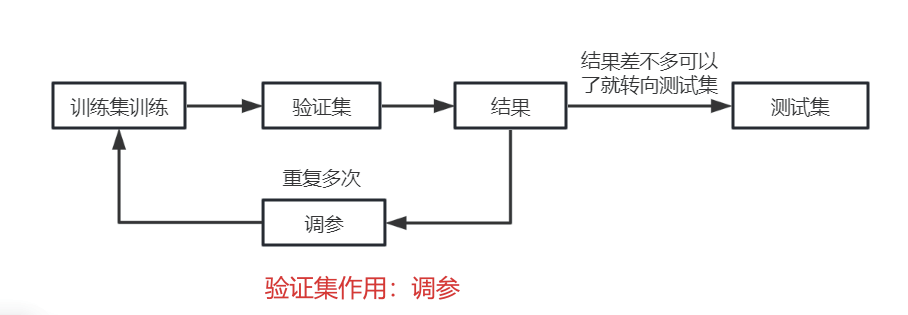## 2.3 性能度量

1、回归任务最常用的性能度量是“均方误差”(mean squared error)

$mean\space squared\space error \space 均方误差： \begin{cases} E(f;D)=\frac{1}{m}\sum_{i=1}^{m}(f(x_{i})-y_{i})^{2},\space\space （2.2） \\ 对于数据分布D和概率密度函数p(·),均方误差可描述为： \\ E(f;D)=\int_{x\in D}(f(x)-y)^{2}p(x)dx.\space\space (2.3) \end{cases}$

### 1.错误率与精度

对样例集$$D=\{(x_{1},y_{1}),...,(x_{m},y_{m})\}$$来说，
错误率

$E(f;D)=\frac{1}{m} \sum_{i=1}^{m}\mathbb{I}(f(x_{i})\neq y_{i})$

$$\mathbb{I}$$(.)是指示函数，若·为真则取值1，否则取值0.

精度

\begin{align} acc(f;D)=&\frac{1}{m} \sum_{i=1}^{m}\mathbb{I}(f(x_{i})= y_{i}) \\=&1-E(f;D) \end{align}

### 2.查准率、查全率和F1

TP：真正例；FP：假正例；TN：真反例；FN：假反例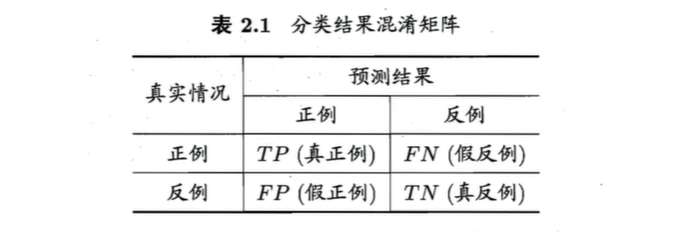#### 查准率Percision

$P=\frac{TP}{TP+FP}$

#### 查全率Recall

$R=\frac{TP}{TP+FN}$

#### P-R曲线

1、混淆矩阵：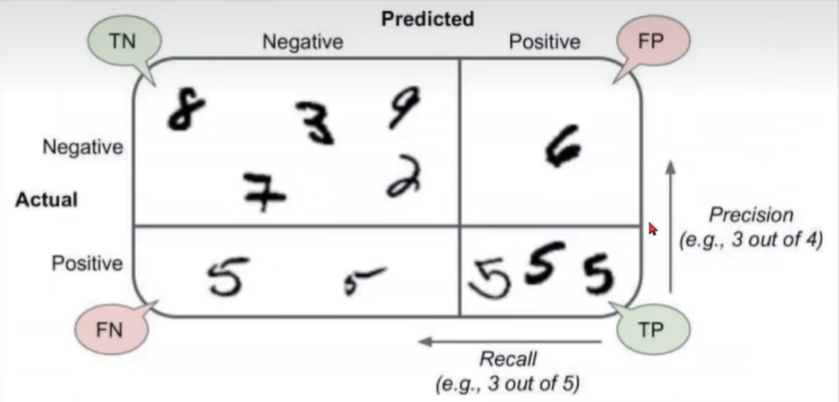2、P-R反向变动关系原理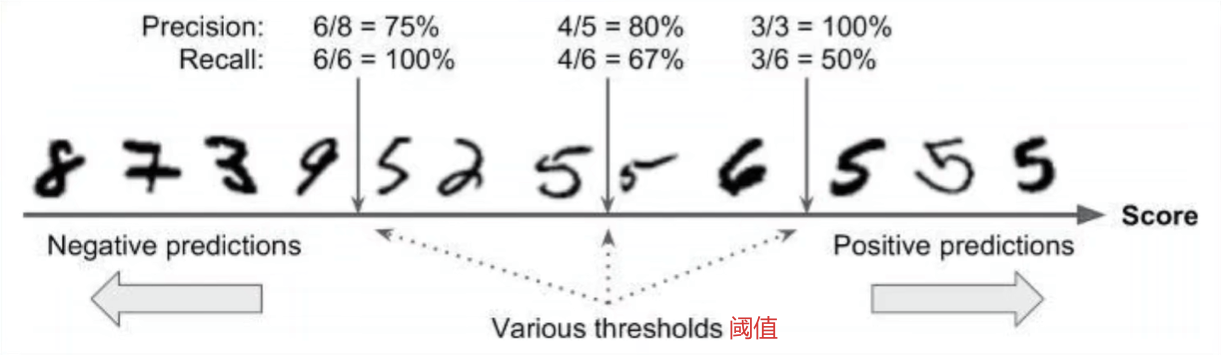3、阈值-PR图像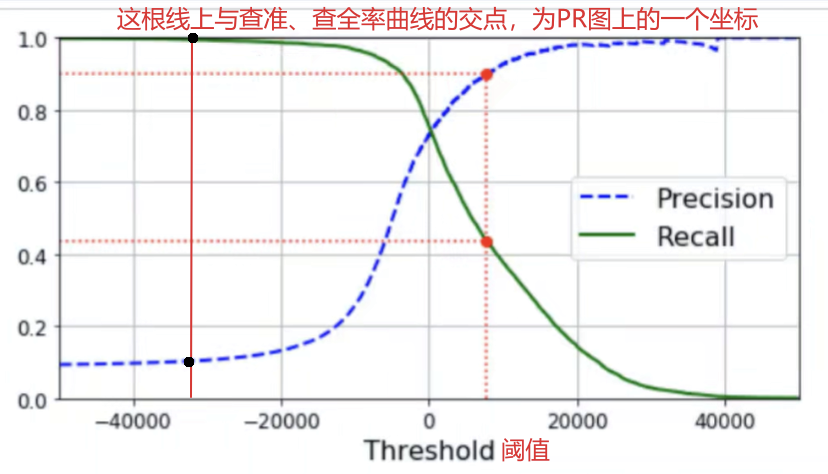4、P-R图像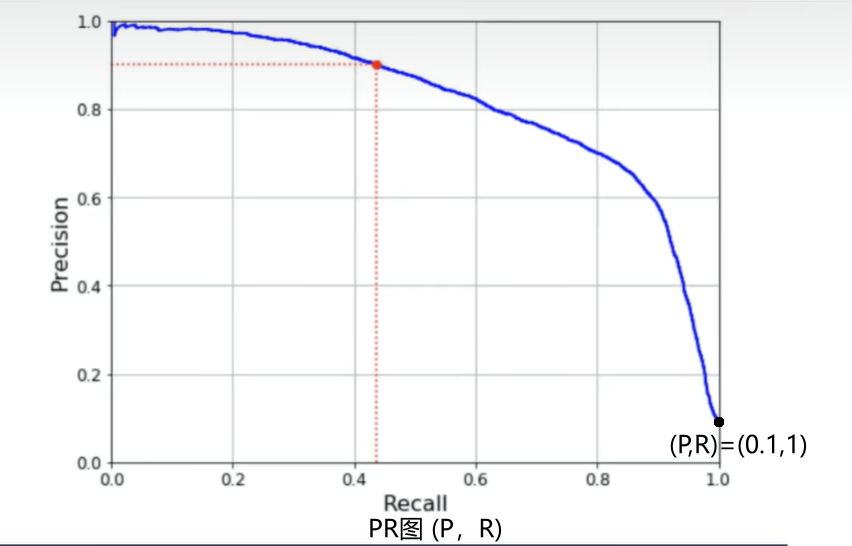5、最优阈值的确定

• 方法一：使用平衡点Break-Even Point(BEP):P=R时，P与R的值
• 方法二：F1度量
• 方法三：$$F_{β}$$度量
• 注意部分

PR图像中，P=R直线与曲线相交点

$$P=\frac{1}{1+3}$$,$$R=\frac{1}{1+2}$$

\begin{align} \frac{1}{F_{1}}=&\frac{1}{2}(\frac{1}{P}+\frac{1}{R}) \\ =&\frac{1}{2}(\frac{1+3}{1}+\frac{1+2}{1}) \\ =&\frac{1}{2}(\frac{(1+3)+(1+2)}{1}) \\ F_{1}=&\frac{1}{(1+3)+(1+2)} \end{align}

\begin{align} \frac{1}{F_{β}}=&\frac{1}{1+β^{2}}(\frac{1}{P}+\frac{β^{2}}{R}) \\ =&\frac{1}{1+β^{2}}(\frac{1+3}{1}+\frac{β^{2}(1+2)}{1}) \\ =&\frac{1}{1+β^{2}}(\frac{(1+3)+β^{2}(1+2)}{1}) \\ F_{β}=&\frac{1+β^{2}}{(1+3)+β^{2}(1+2)} \end{align}

$$β=1$$时退化为标准的$$F_{1}$$;
$$β>1$$时查全率有更大的影响；
$$β<1$$时查准率有更大的影响。

$$F_{1}$$是基于查准率与查全率的调和平均（harmonic mean）定义的：

$\frac{1}{F_{1}}=\frac{1}{2}(\frac{1}{P}+\frac{1}{R})$

$$F_{β}$$则是加权调和平均：

$\frac{1}{F_{β}}=\frac{1}{1+β^{2}}(\frac{1}{P}+\frac{β^{2}}{R})$

#### n个二分类实现的多分类问题

• 先分别计算，再求平均值
• 先平均在计算

1、先分别计算，再求平均值

$$(P_{1},R_{1}),(P_{2},R_{2}),(P_{3},R_{3})...,(P_{n},R_{n})$$

$macro_P宏查准率：macro_P=\frac{1}{n}\sum_{i=1}^{n}P_{i}$

$macro_R宏查全率：macro_R=\frac{1}{n}\sum_{i=1}^{n}R_{i}$

$macro_{F_1}宏F_{1}:macro_{F_{1}}=\frac{2×macro_P×macro_R}{macro_P+macro_R}$

2、先求平均值再计算

$$\overline{TP},\overline{FP},\overline{TN},\overline{FN}$$

$micro_P宏查准率：micro_P=\frac{\overline{TP}}{\overline{TP}+\overline{FP}}$

$micro_R宏查全率：micro_R=\frac{\overline{TP}}{\overline{TP}+\overline{FN}}$

$micro_{F_1}宏F_{1}:micro_{F_{1}}=\frac{2×micro_P×micro_R}{micro_P+micro_R}$

### 3.ROC与AUC

ROC曲线的纵轴是真正率(TPR),横轴是假正率(FPR)

$TPR=\frac{TP}{TP+FN}或\frac{①}{①+②} (TP在横向中占比) \\ \\ FPR=\frac{FP}{TN+FP}或\frac{③}{③+④}（FP在横向中占比）$

T TP(真正例) ① FN(假反例) ②
F FP(假正例) ③ TN(真反例) ④

ROC曲线下分析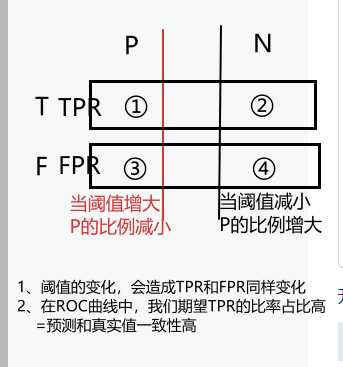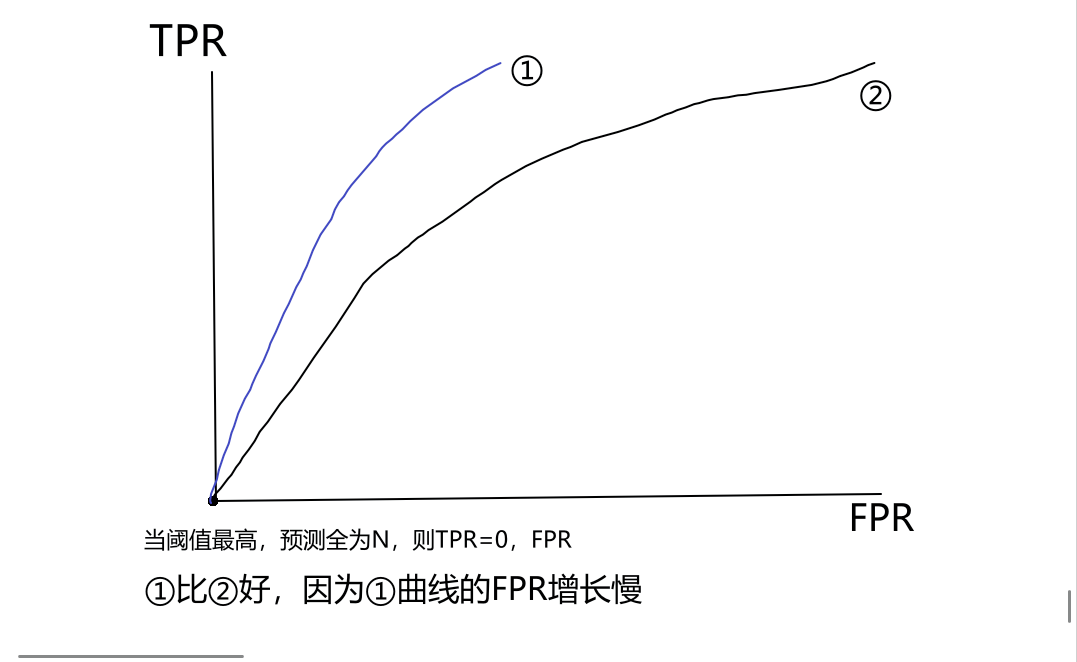ROC示意图：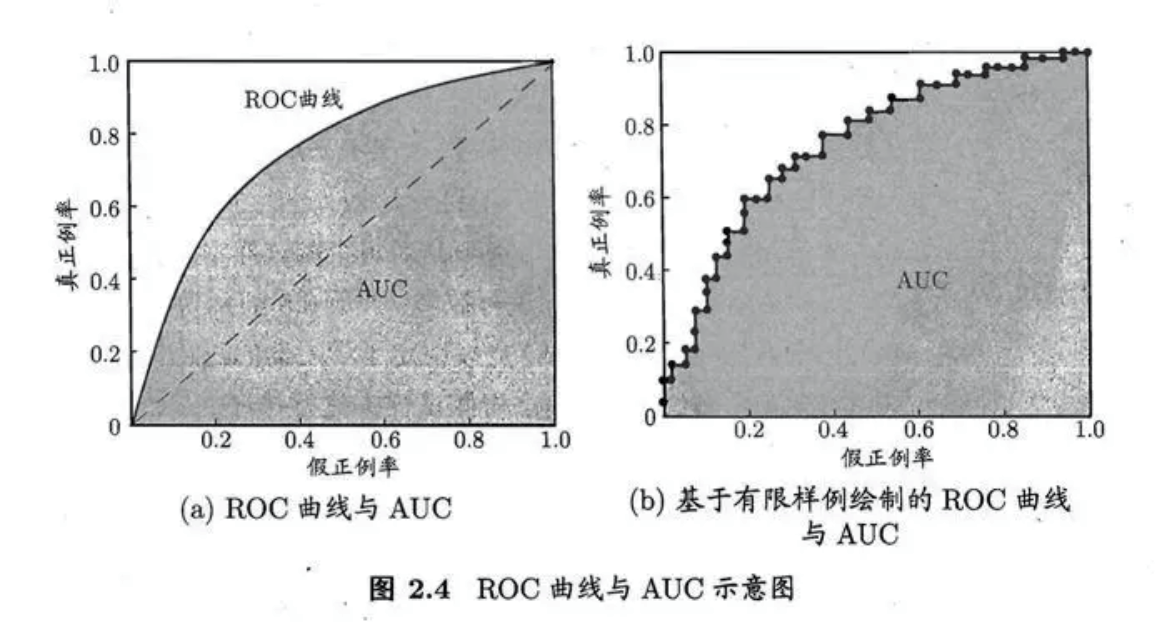2、AUC(Area under ROC Curve)：ROC曲线下的面积

$AUC=\frac{1}{2}\sum_{i=1}^{m-1}(x_{i+1}-x_{i})(y_{i}+y_{i+1})$

$l_{rank}=\frac{1}{m^{+}m^{-}}\sum_{x^{+}\in D^{+}}\sum_{x^{-}\in D^{-}}(\mathbb{I}\{f(x^{+})<f(x^{-}\}+\frac{1}{2}\mathbb{I}\{f(x^{+})=f(x^{-}\})$

$$l_{rank}计算$$举例分析：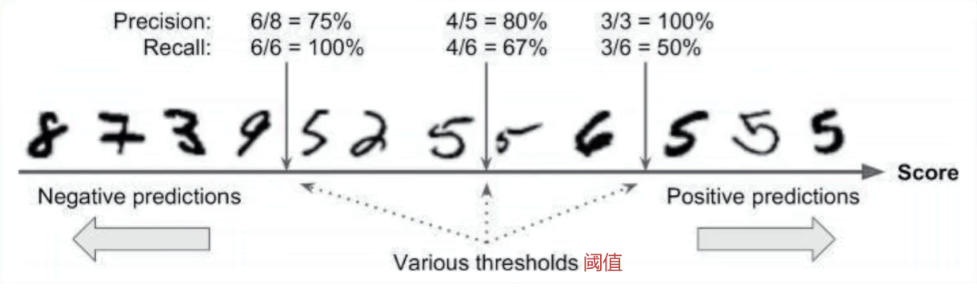D(正反例集合) m(正例、反例) P N
+ D+(正例集合)[5,5,5,5,5,5] m+(6个)
- D-(反例例集合)[8,7,3,9,2,6] m-(6个)

$$l_{rank}$$计算过程：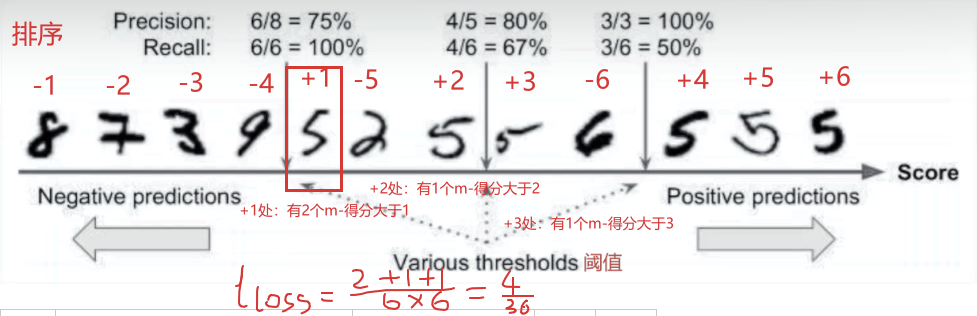考虑每一对正反例，若正例的预测值小于反例，则记一个“罚分”，若相等，则记0.5个“罚分”。容易看出，$$l_{rank}$$对应的是ROC曲线上的面积：一个正例在ROC曲线上对应标记点的坐标是(x,y)则x恰是排序在其之前的反例所占的比例，即假正例率.因此有：

$AUC=1-l_{rank}$

AUC公式分析：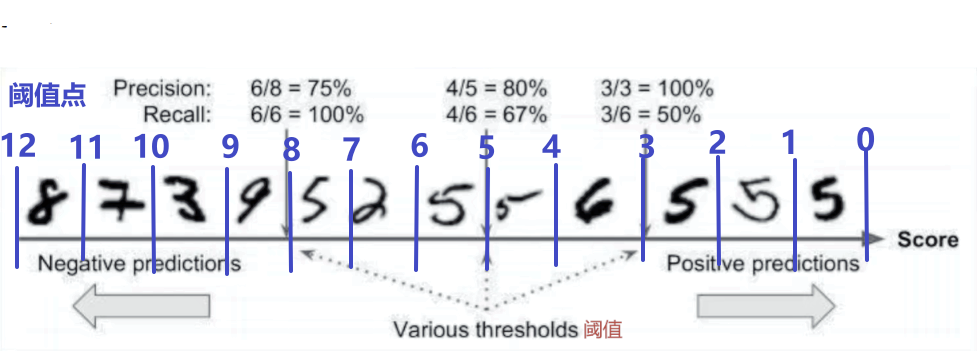ROC\阈值点 0 1 2 3 4 5 6 7 8 9 10 11 12
TPR 0/6 1/6 2/6 3/6 3/6 4/6 5/6 5/6 6/6 6/6 6/6 6/6 6/6
FPR 0/6 0/6 0/6 0/6 1/6 1/6 1/6 2/6 2/6 3/6 4/6 5/6 6/6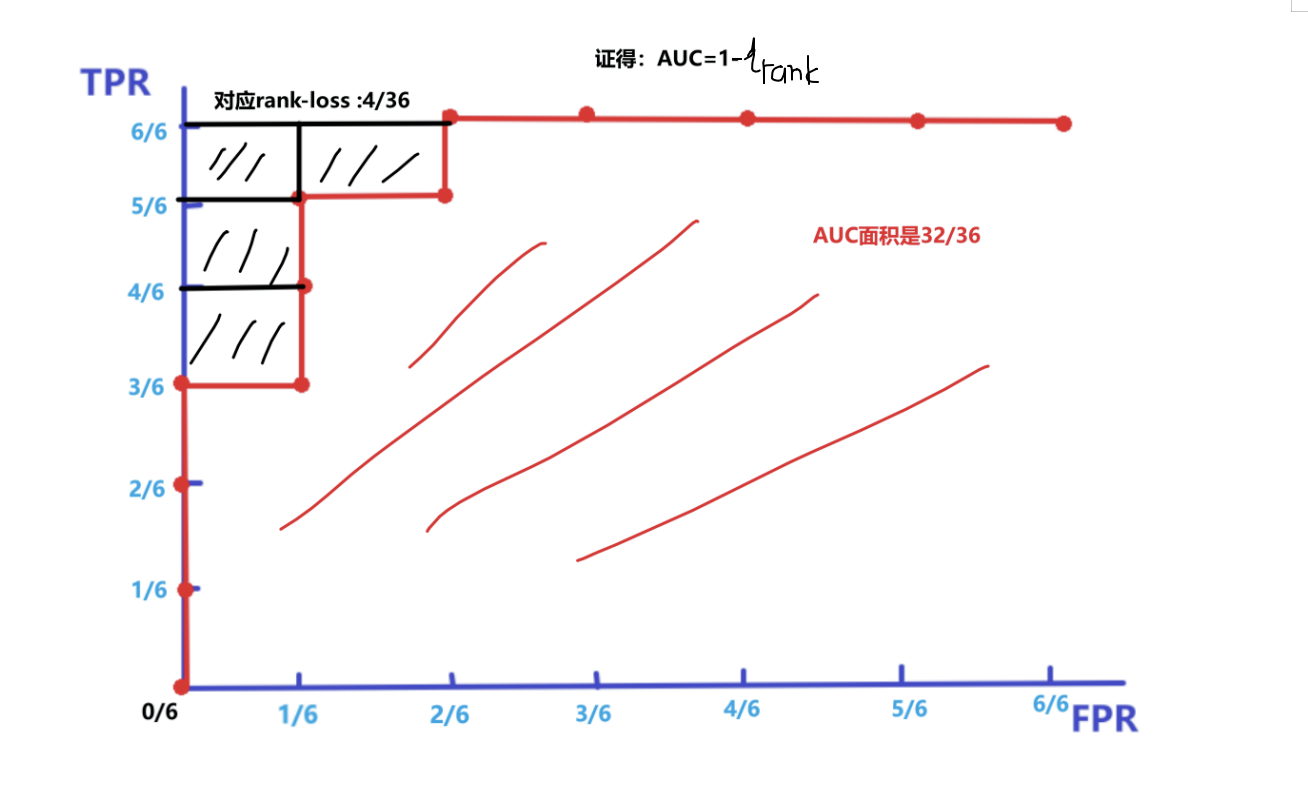### 4.代价敏感错误率与代价曲线

1、为权衡不同类型错误所造成的不同损失，可为错误赋予“非均等代价”(unequal cost)
2、在非均等代价下，我们所希望的不再是简单地最小化错误次数，而是希望最小化“总体代价”(total cost)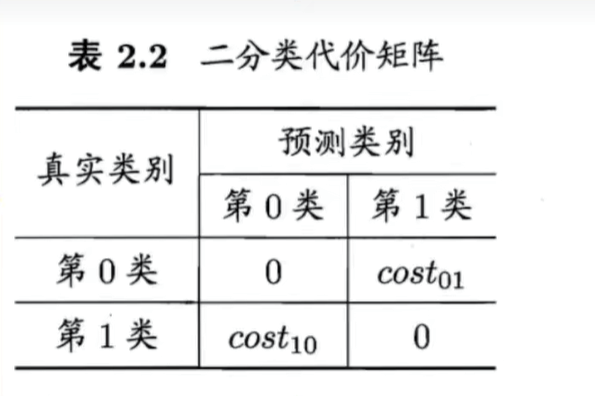“代价敏感”错误率(Error)为

$E(f;D;cost)=\frac{1}{m}(\sum_{x_{i}\in D^{+}} \mathbb{I}(f(x_{i})\neq y_{i})×cost_{01}+\sum_{x_{i}\in D^{-}} \mathbb{I}(f(x_{i})\neq y_{i})×cost_{10})$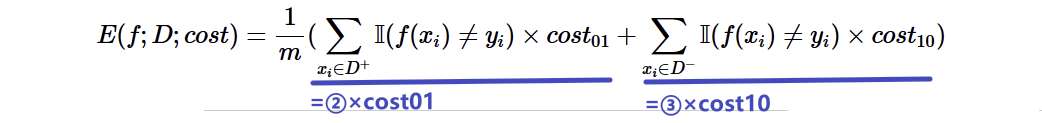1、明确参数：$$p=\frac{m_{+}}{m}$$(正例占比)
2、代价曲线基本思路：

$P(+)cost=\frac{p×cost_{01}}{p×cost_{01}+(1-p)×cost_{10}} \\ p=0,P(+)cost=0 \\ p=1,p(+)cost=1$

$cost_{norm}=\frac{FNR×p×cost_{01}+FPR×(1-p)×cost_{10}}{p×cost_{01}+(1-p)×cost_{10}} \\ p=0,cost_{norm}=FPR \\ p=1,cost_{norm}=FNR$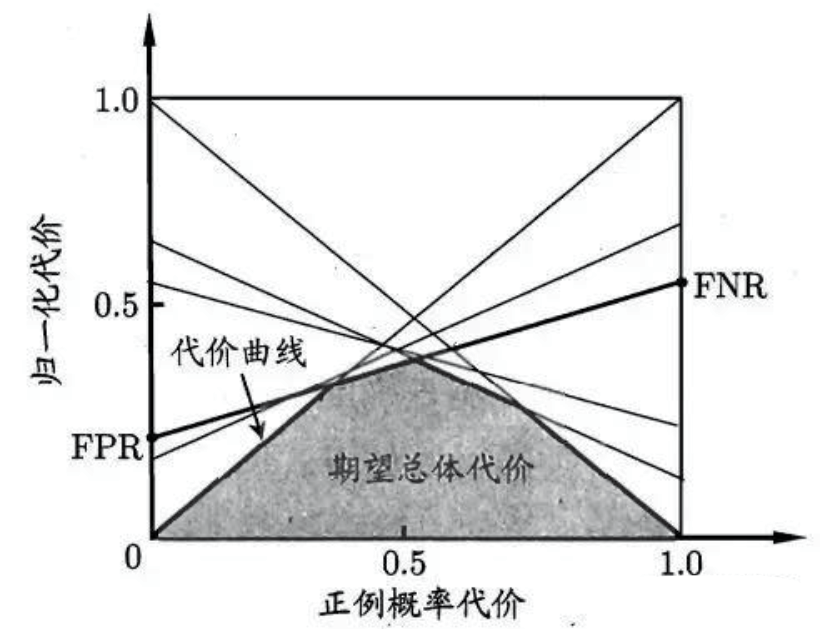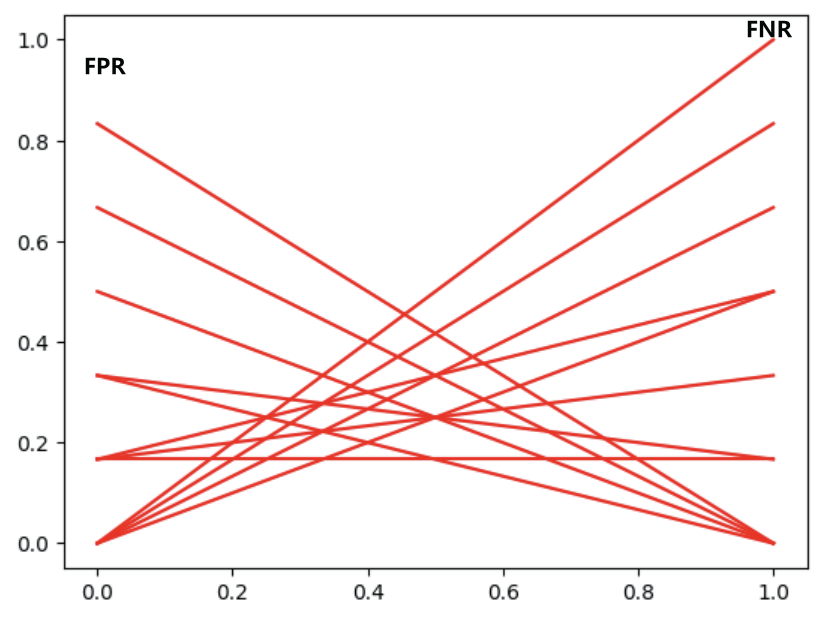## 2.4比较检验

### 1、二项检验

1、测试集上的性能与真正的泛化性能未必相同
2、测试集上的性能和测试集本身有很大关系，即使使用相同测试集，测试的结果也会有不同。
3、很多机器学习算法本身具有一定的随机性，即便用相同的参数设置在同一测试集上，其结果也会不同。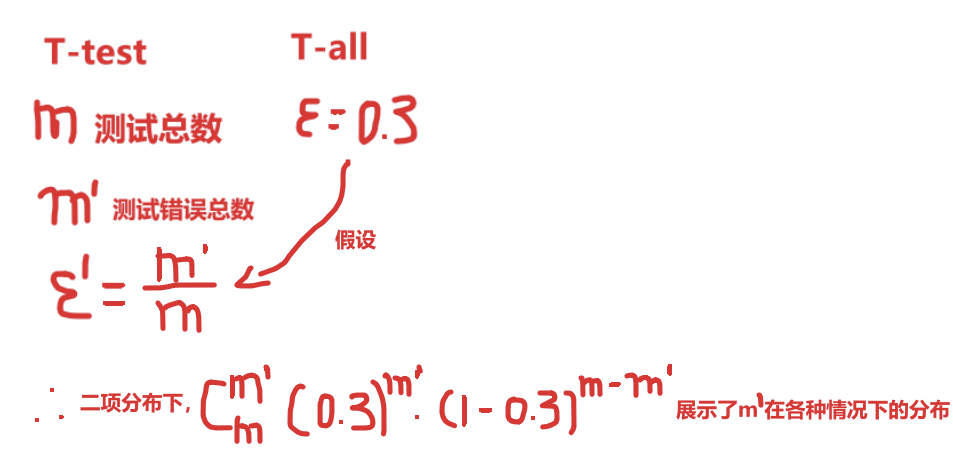$$即：P(\hat{\epsilon};\epsilon)=(\begin{matrix}m\\\hat{\epsilon×m}\end{matrix})\epsilon^{\hat{\epsilon}×m}(1-\epsilon)^{m-\hat{\epsilon}×m}$$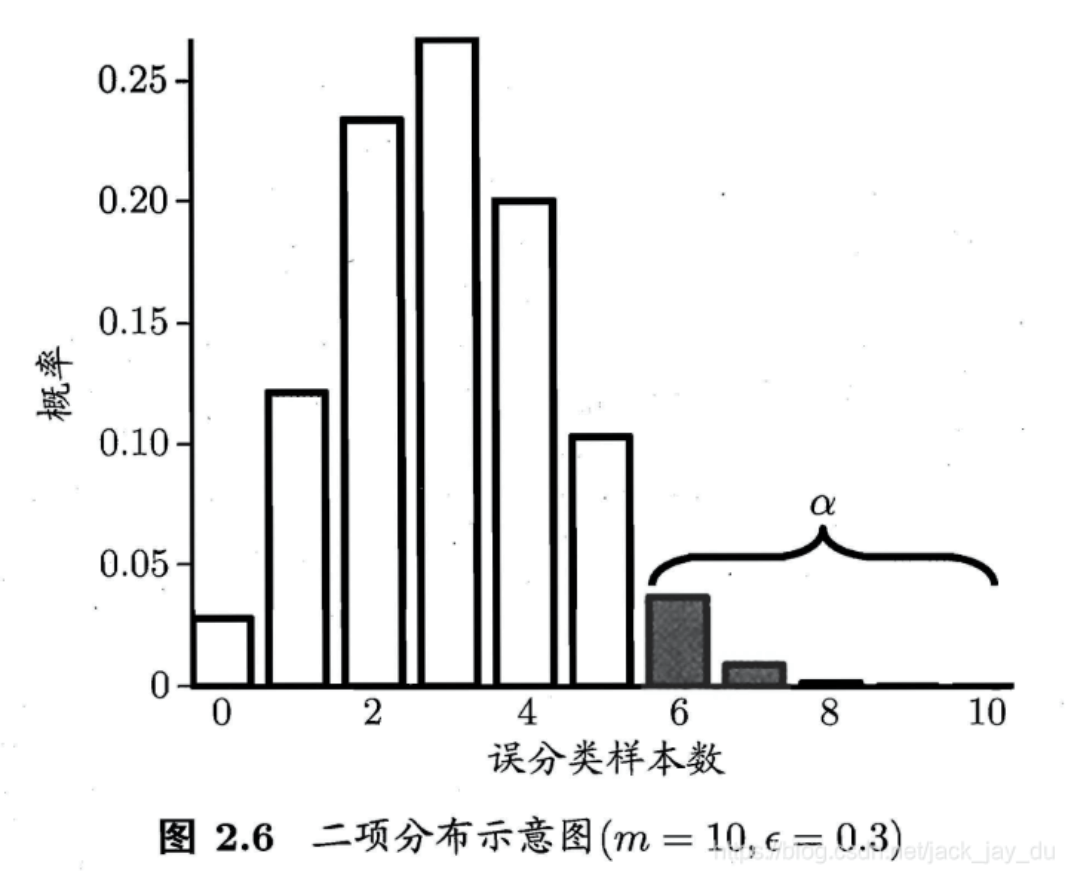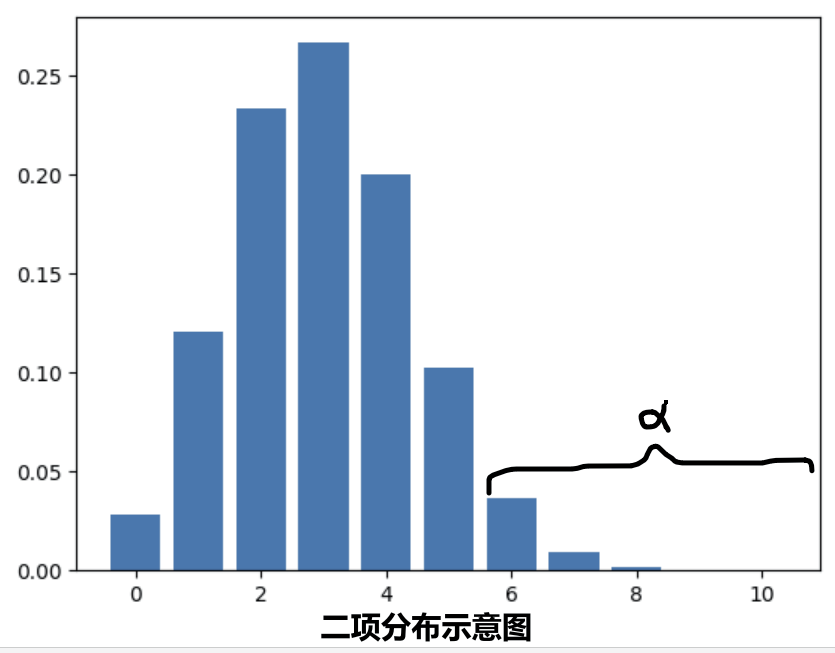$$\alpha$$：不可置信度

$\overline{\epsilon}=max(P(\hat{\epsilon},\epsilon))<\alpha$

假设泛化错误率为$$\epsilon \leq \epsilon_{0}$$,在测试集样本中，以泛化错误率为测试集错误率，对测试集不同错误数的情况检验其概率，得到二项分布图，当真实测试集的错误样本数在$$1-\alpha$$区域内，则称有$$1-\alpha$$的置信度说，泛化错误率小于$$\epsilon_{0}$$

### 2、t检验

$\mu=\frac{1}{k}\sum_{i=1}^{k}\hat{\epsilon_{i}}$

$\sigma^{2}=\frac{1}{k-1}\sum_{i=1}^{k}(\hat{\epsilon}_{i}-\mu)^{2}$

## 小结：假设检验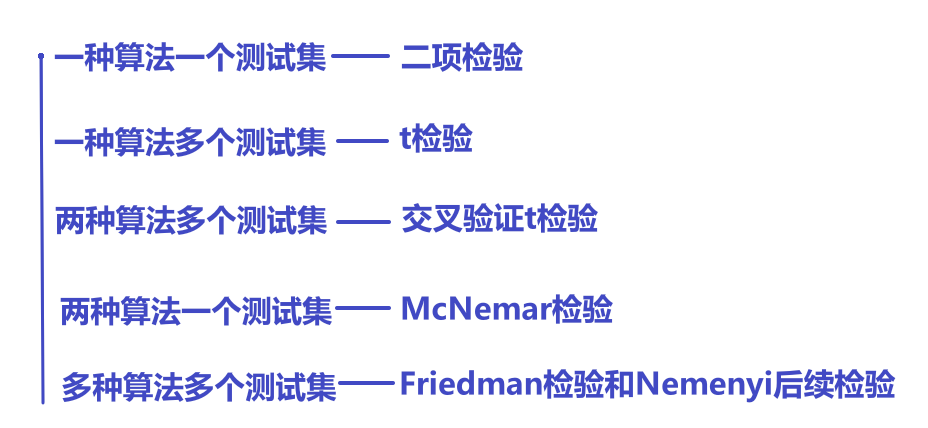下一章：3-4

posted @ 2023-10-03 23:53  AlexanderOscar  阅读(63)  评论(0编辑  收藏  举报